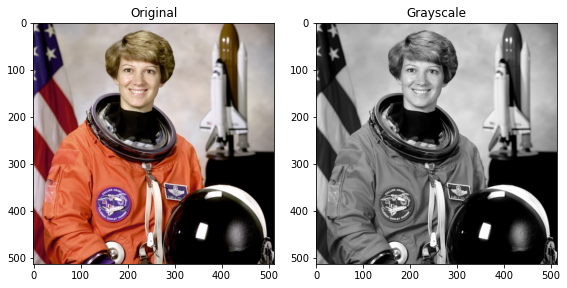# 19. RGB to gray¶

This example converts an image with RGB channels into an image with a single grayscale channel.

The value of each grayscale pixel is calculated as the weighted sum of the corresponding red, green and blue pixels as:

Y = 0.2125 R + 0.7154 G + 0.0721 B

These weights are used by CRT phosphors as they better represent human perception of red, green and blue than equal weights.

import numpy as np
import matplotlib.pyplot as plt

from skimage import data
from skimage.color import rgb2gray


## 19.1. Load the original image and apply the rgb2gray function¶

original = data.astronaut()
grayscale = rgb2gray(original)


## 19.2. Show the images side by side¶

fig, axes = plt.subplots(1, 2, figsize=(8, 4))
ax = axes.ravel()

ax.imshow(original)
ax.set_title("Original")
ax.imshow(grayscale, cmap=plt.cm.gray)
ax.set_title("Grayscale")

fig.tight_layout()
plt.show()